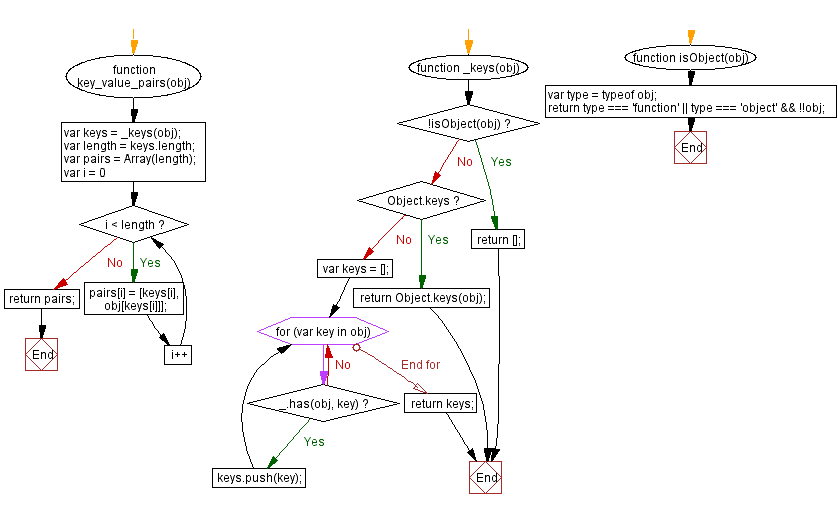# JavaScript: Convert an object into a list of pairs

## JavaScript Object: Exercise-15 with Solution

Write a JavaScript function to convert an object into a list of '[key, value]' pairs.

Sample Solution: -

HTML Code:

``````<!DOCTYPE html>
<html>
<meta charset="utf-8">
<title>JavaScript function to convert an object into a list of pairs.</title>
<body>

</body>
</html>
```
```

JavaScript Code:

``````function key_value_pairs(obj)
{
var keys = _keys(obj);
var length = keys.length;
var pairs = Array(length);
for (var i = 0; i < length; i++)
{
pairs[i] = [keys[i], obj[keys[i]]];
}
return pairs;
}

function _keys(obj)
{
if (!isObject(obj)) return [];
if (Object.keys) return Object.keys(obj);
var keys = [];
for (var key in obj) if (_.has(obj, key)) keys.push(key);
return keys;
}
function isObject(obj)
{
var type = typeof obj;
return type === 'function' || type === 'object' && !!obj;
}
console.log(key_value_pairs({red: "#FF0000", green: "#00FF00", white: "#FFFFFF"}));
```
```

Sample Output:

```[["red","#FF0000"],["green","#00FF00"],["white","#FFFFFF"]]
```

Flowchart:Live Demo:

See the Pen javascript-object-exercise-15 by w3resource (@w3resource) on CodePen.

Improve this sample solution and post your code through Disqus

What is the difficulty level of this exercise?

﻿

## JavaScript: Tips of the Day

objects interact by reference

```let c = { greeting: 'Hey!' };
let d;

d = c;
c.greeting = 'Hello';
console.log(d.greeting);
```

In JavaScript, all objects interact by reference when setting them equal to each other.

First, variable c holds a value to an object. Later, we assign d with the same reference that c has to the object.

When you change one object, you change all of them.

Ref: https://bit.ly/323Y0P6